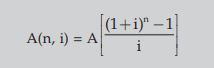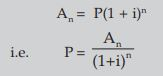# CA Foundation Time Value of Money Summary

## CA Foundation Time Value of Money Summary Applicable Upcoming Exams.

• Time value of money: Time value of money means that the value of a unity of money is different in different time periods. The sum of money received in future is less valuable than it is today. In other words the present worth of money received after some time will be less than a money received today.
• Interest: Interest is the price paid by a borrower for the use of a lender’s money. If you borrow (or lend) some money from (or to) a person for a particular period you would pay (or receive) more money than your initial borrowing (or lending).
• Simple interest: is the interest computed on the principal for the entire period of borrowing.

I = Pit
A = P + I
I = A – P
Here, A = Accumulated amount (final value of an investment)
P = Principal (initial value of an investment)
i = Annual interest rate in decimal.
I = Amount of Interest
t = Time in years

• Compound interest as the interest that accrues when earnings for each specified period of time added to the principal thus increasing the principal base on which subsequent interest is computed.

Formula for compound interest:
An = P ( 1 + i)n
where, i = Annual rate of interest
n = Number of conversion periods per year
Interest = An – P = P ( 1 + i )n – P
n is total conversions i.e. t x no. of conversions per year

• Effective Rate of Interest: The effective interest rate can be computed directly by following formula:

E = (1 + i)n– 1
Where E is the effective interest rate
i = actual interest rate in decimal
n = number of conversion period

• Future value of a single cash flow can be computed by above formula. Replace A by future value (F) and P by single cash flow (C.F.) therefore

F = C.F. (1 + i)n

• Annuity can be defined as a sequence of periodic payments (or receipts) regularly over a specified period of time.

Annuity may be of two types:
(i) Annuity regular: In annuity regular first payment/receipt takes place at the end of first period.
(ii) Annuity Due or Annuity Immediate: When the first receipt or payment is made today (at the beginning of the annuity) it is called annuity due or annuity immediate

• If A be the periodic payments, the future value A(n, i) of the annuity is given by• Future value of an Annuity due/Annuity immediate = Future value of annuity regular x (1+i) where i is the interest rate in decimal.
• The present value P of the amount An due at the end of n period at the rate of i per interest period may be obtained by solving for P the below given equation• Present value of annuity due or annuity immediate: Present value of annuity due/ immediate for n years is the same as an annuity regular for (n-1) years plus an initial receipt or payment in beginning of the period. Calculating the present value of annuity due involves two steps.

Step 1: Compute the present value of annuity as if it were a annuity regular for one period short.
Step 2: Add initial cash payment/receipt to the step 1 value.

• Sinking Fund: It is the fund credited for a specified purpose by way of sequence of periodic payments over a time period at a specified interest rate. Interest is compounded at the end of every period. Sizeof the sinking fund deposit is computed from A = P.A(n,i) where A is the amount to be saved the periodic payment, n the payment period.
• Annuity applications:

(a) Leasing: Leasing is a financial arrangement under which the owner of the asset (lessor) allows the user of the asset (lessee) to use the asset for a defined period of time (lease period) for a consideration (lease rental) payable over a given period of time. This is a kind of taking an asset on rent.

(b) Capital Expenditure (investment decision): Capital expenditure means purchasing an asset (which results in outflows of money) today in anticipation of benefits (cash inflow) which would flow across the life of the investment.

(c) Valuation of Bond: A bond is a debt security in which the issuer owes the holder a debt and is obliged to repay the principal and interest. Bonds are generally issued for a fixed term longer than one year.